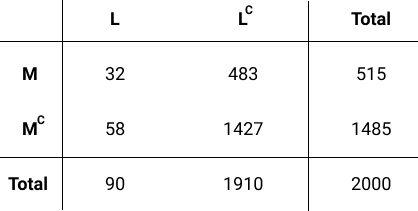# Conditional Probability: dependent events - where is the logic?

I am trying to understand the logic of the exercises where we need to solve two cases based on the table:Find whether the following events are independent or not (check the hint if you don’t know how to solve this):

1. Events L and M — assign the string ‘independent’ to a variable named `l_and_m` if the events are independent, otherwise assign the string ‘dependent’.
2. Events L and MC — assign the string ‘independent’ to a variable named `l_and_non_m` if the events are independent, otherwise assign the string 'dependent

If I think logically about the condition those events should be independent - a customer is buying a mouse (M) or not buying, a customer is buying (L) or not buying a laptop. This is the same scenario as if we are rolling a a dice two times, both outcomes will be random and independent.

Why are we saying then that L and M are dependent events? Unless this is an absolutely theoretical example which has nothing to do with the reality.

I suspect I might be misunderstanding the meaning of “independent” in the context of the lesson.

…if event A occurs and the probability of B remains unchanged and vice versa (A and B can be any events for any random experiment), then events A and B are said to be statistically independent

Does the above statement and the table remove “randomness” and hence the probability of L changes because M occurred?

Could someone explain to me please?

Not sure what you mean a statement or table removing randomness. Whether independent or not, randomness is there. Random variable just means a variable that can have varying outcome values from a random experiment.

If we want to work with probability theory, or any math generally, we have to follow their axioms. If P(A|B) = P(A), then A and B are independent. Doesn’t matter what your worldview is or what you observe of the world.
Theories exist because they are correct as an abstraction enough times to be practically useful even if not always matching experimental observations. For example the probability of a fair coin giving heads is said to be 50% even though your flips may show H,H,H,T, or if you only flip 3 times and don’t allow a 50% result to show no matter what.

In this example, the probability of L changed when M is considered, so L and M are not independent, which of course also means L and M_complement are not independent.

1 Like

Ok, thank you, I think I got my answer - this is purely theoretical and has nothing to do with the real world.
I think the confusion was because of the example - rolling a dice is a clear example of probability, laptops and mouse purchases was not, at least for me.